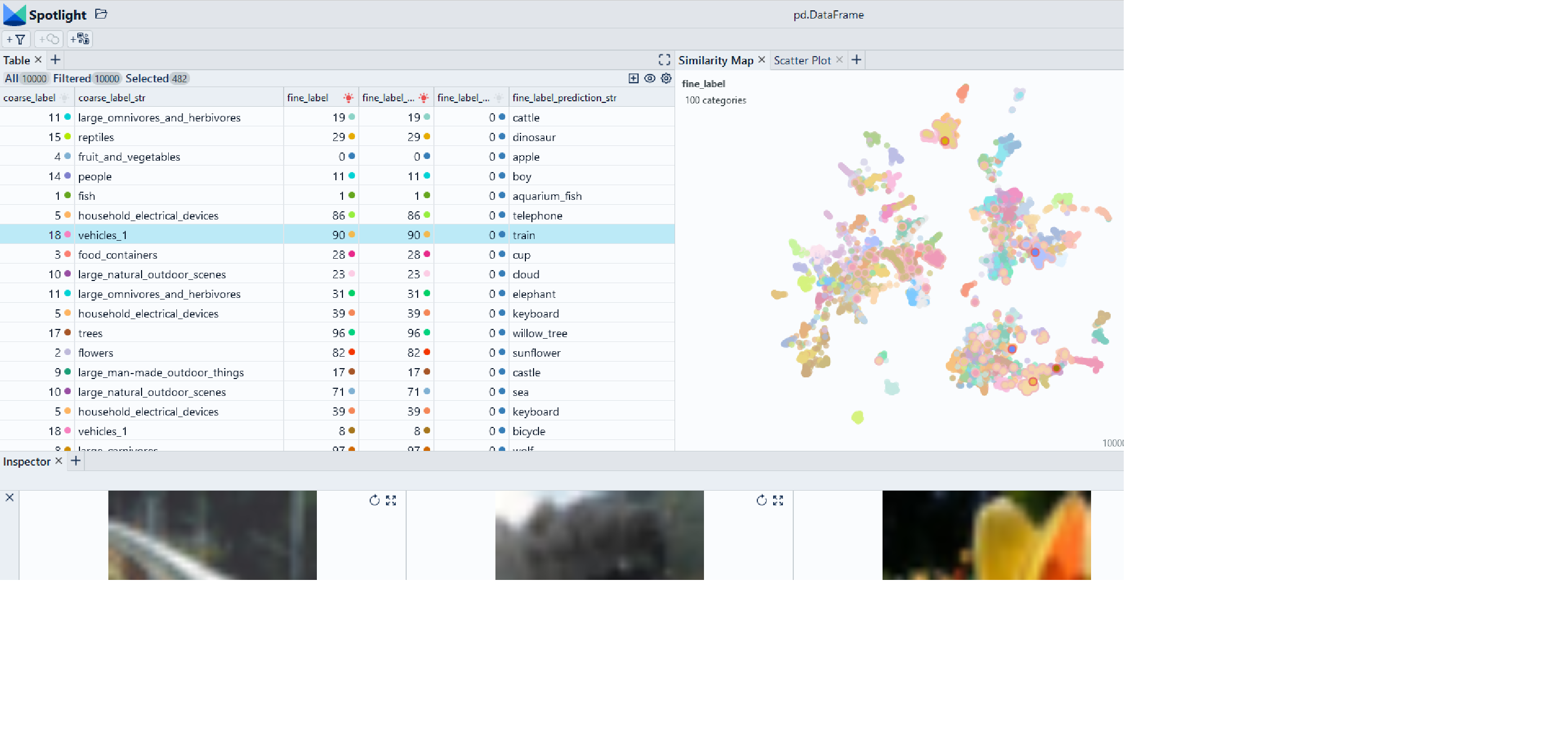🎉 We released Spotlight 1.4.0 check it out

Version: 1.1.0

# Create image embeddings with Huggingface

We use the Huggingface transformers library to create an embedding for a an image dataset.

Use Chrome to run Spotlight in Colab. Due to Colab restrictions (e.g. no websocket support), the performance is limited. Run the notebook locally for the full Spotlight experience.• `df['image']` contains the paths to the images in the dataset## Imports and play as copy-n-paste functions​

# Install dependencies
``# Imports!pip install renumics-spotlight transformers torch datasets``
# Play as copy-n-paste functions
``import datasetsfrom transformers import AutoFeatureExtractor, AutoModelimport torchfrom renumics import spotlightimport pandas as pddef extract_embeddings(model, feature_extractor, image_name='image'):    """Utility to compute embeddings."""    device = model.device    def pp(batch):        images = batch["image"]        inputs = feature_extractor(images=images, return_tensors="pt").to(device)        embeddings = model(**inputs).last_hidden_state[:, 0].cpu()        return {"embedding": embeddings}    return ppdef huggingface_embedding(df, image_name='image', inplace=False, modelname='google/vit-base-patch16-224', batched=True, batch_size=24):    # initialize huggingface model    feature_extractor = AutoFeatureExtractor.from_pretrained(modelname)    model = AutoModel.from_pretrained(modelname, output_hidden_states=True)    # create huggingface dataset from df    dataset = datasets.Dataset.from_pandas(df).cast_column(image_name, datasets.Image())    #compute embedding    device = "cuda" if torch.cuda.is_available() else "cpu"    extract_fn = extract_embeddings( model.to(device), feature_extractor,image_name)    updated_dataset = dataset.map(extract_fn, batched=batched, batch_size=batch_size)    df_temp = updated_dataset.to_pandas()    if inplace:        df['embedding']=df_temp['embedding']        return    df_emb = pd.DataFrame()    df_emb['embedding'] = df_temp['embedding']    return df_emb``

## Step-by-step example on CIFAR-100​

### Load CIFAR-100 from Huggingface hub and convert it to Pandas dataframe​

``dataset = datasets.load_dataset("renumics/cifar100-enriched", split="train")df = dataset.to_pandas()``

### Compute embedding with vision transformer from Huggingface​

``df_emb = huggingface_embedding(df, modelname="google/vit-base-patch16-224")df = pd.concat([df, df_emb], axis=1)``

### Reduce embeddings for faster visualization​

``import umapimport numpy as npembeddings = np.stack(df['embedding'].to_numpy())reducer = umap.UMAP()reduced_embedding = reducer.fit_transform(embeddings)df['embedding_reduced'] = np.array(reduced_embedding).tolist()``

### Perform EDA with Spotlight​

``df_show = df.drop(columns=['embedding', 'probabilities'])spotlight.show(df_show, port=port, dtype={"image": spotlight.Image, "embedding_reduced": spotlight.Embedding})``